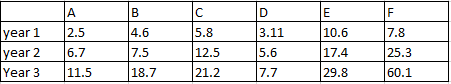### CAT 1996 Question Paper Question 3

Instructions

Direction for questions: Answer the questions based on the following information. The data given in the table shows the investment details in country ‘Fortune Land’ of companies A, B, C, D, E and F. Figures in the table are in US dollars in billions.Question 3

# What is D’s contribution as a percentage of total investments in year 2?

Solution

Total contribution in year 2 = 6.7 + 7.5 + 12.5 + 5.6 +  17.4 + 25.3 = 75 billion

Contibution by D in year 2 = 5.6

% contribution by D = $$\frac{5.6}{75}*100$$ = 7.4%